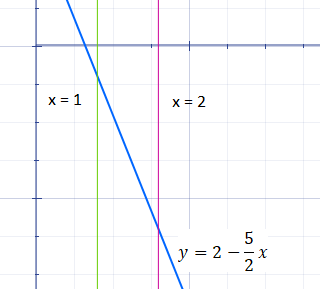# Find the volume V of the solid obtained by rotating the region bounded by the given curves about...

## Question:

Find the volume {eq}V {/eq} of the solid obtained by rotating the region bounded by the given curves about the {eq}x {/eq}-axis.

{eq}y = 2 - \frac{5}{2} x, \; y = 0, \; x = 1, \; x = 2 {/eq}

## Volume of a Solid of Revolution

In this problem we are asked to find the volume of a solid created by rotating a bounded region about the x axis. We will use the formula for volume generated by rotating about the x-axis:

{eq}V = \pi\int_{a}^{b}y^{2}dx{/eq}

## Answer and Explanation:

The solid is created by rotating the bounded region shown in the graph around the x-axis.First let's set up our equation for volume using the formula. The x-values for our region are from 1 to 2.

{eq}V = \pi\int_{a}^{b}y^{2}dx = \pi\int_{1}^{2}(2-\frac{5}{2}x)^{2}dx\\ V = \pi\int_{1}^{2}(4-10x+\frac{25x^2}{4})dx {/eq}

Now we can integrate and evaluate to find the volume.

{eq}V = \pi(4x-5x^2+\frac{25x^3}{12})|_{1}^{2}\\ V = \pi((4(2)-5(2)^2+\frac{25(2)^3}{12})-(4-5+\frac{25}{12}))\\ V = \frac{43\pi}{12}\approx 11.26 {/eq}